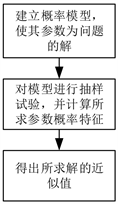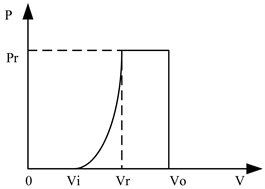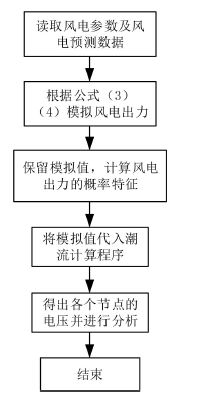# 基于蒙特卡罗方法的风电场潮流计算Wind Power Flow Calculation Based on Monte Carlo Method

DOI: 10.12677/JEE.2019.73018, PDF, HTML, XML, 下载: 304  浏览: 758  科研立项经费支持

Abstract: With the rapid development of wind energy, wind power can be integrated into the power grid on a large scale, which exacerbates the uncertainty of power system operation, making the power flow calculation of traditional power systems insufficient to adapt to many current uncertain factors. In view of this situation, this paper proposes the power flow calculation of uncertain power system based on Monte Carlo method. Firstly, based on the uncertainty characteristics of wind power, the mathematical model and probability distribution of wind power output are established, and then the wind power system with suitable power flow calculation is constructed. Secondly, according to the data sample, qualified injection nodes are found on the IEEE14 node power distribution system. Finally, the Newton-Raphson method is used to calculate the current of the system with wind power generation. The numerical results show that the method presented in this paper is more accurate in calculating the power flow of the system and can meet the engineering requirements.

1. 引言

2. 蒙特卡罗法

${X}_{N}=\frac{1}{N}\underset{n=1}{\overset{N}{\sum }}{x}_{n}$ (1)

$P\left(\underset{N\to \infty }{\mathrm{lim}}{X}_{N}=\omega \right)=1$ (2)Figure 1. The basic idea of montecarlo

3. 风能模型

3.1. 风速概率模型

$f\left(v\right)=\left(\frac{k}{c}\right){\left(\frac{v}{c}\right)}^{k-1}\mathrm{exp}\left[-{\left(\frac{v}{c}\right)}^{k}\right]$ (3)

$F\left(v\right)=1-\mathrm{exp}\left[-{\left(\frac{v}{c}\right)}^{k}\right]$ (4)

3.2. 风力发电机输出模型Figure 2. Wind turbine output power curve

$P=\left\{\begin{array}{ll}0\hfill & \text{\hspace{0.17em}}\text{\hspace{0.17em}}\text{\hspace{0.17em}}\text{ }V\le {V}_{i}\hfill \\ \frac{{P}_{r}}{{V}_{r}^{3}-{V}_{i}^{3}}\left({V}^{3}-{V}_{i}^{3}\right)\hfill & {V}_{i}{V}_{o}\hfill \end{array}$ (5)

4. 牛顿-拉夫逊法潮流计算

${U}_{i}={e}_{i}+j{f}_{i}$ (6)

${Y}_{ij}={G}_{ij}+j{B}_{ij}$ (7)

${P}_{i}={e}_{i}\underset{j=1}{\overset{n}{\sum }}\left({G}_{ij}{e}_{i}-{B}_{ij}{f}_{j}\right)+{f}_{j}\underset{j=1}{\overset{n}{\sum }}\left({G}_{ij}{f}_{j}+{B}_{ij}{e}_{j}\right)$ (8)

${Q}_{i}={f}_{i}\underset{j=1}{\overset{n}{\sum }}\left({G}_{ij}{e}_{i}-{B}_{ij}{f}_{j}\right)-{f}_{j}\underset{j=1}{\overset{n}{\sum }}\left({G}_{ij}{f}_{j}+{B}_{ij}{e}_{j}\right)$ (9)

$\Delta {P}_{i}={P}_{is}-{P}_{i}={P}_{i}{}_{s}-{e}_{i}\underset{j=1}{\overset{n}{\sum }}\left({G}_{ij}{e}_{i}-{B}_{ij}{f}_{j}\right)-{f}_{j}\underset{j=1}{\overset{n}{\sum }}\left({G}_{ij}{f}_{j}+{B}_{ij}{e}_{j}\right)=0$ (10)

$\Delta {Q}_{i}={Q}_{is}-{Q}_{i}={Q}_{i}{}_{s}-{f}_{i}\underset{j=1}{\overset{n}{\sum }}\left({G}_{ij}{e}_{i}-{B}_{ij}{f}_{j}\right)+{e}_{j}\underset{j=1}{\overset{n}{\sum }}\left({G}_{ij}{f}_{j}+{B}_{ij}{e}_{j}\right)=0$ (11)

$\Delta {P}_{i}={P}_{is}-{P}_{i}={P}_{i}{}_{s}-{e}_{i}\underset{j=1}{\overset{n}{\sum }}\left({G}_{ij}{e}_{i}-{B}_{ij}{f}_{j}\right)-{f}_{j}\underset{j=1}{\overset{n}{\sum }}\left({G}_{ij}{f}_{j}+{B}_{ij}{e}_{j}\right)=0$ (12)

$\Delta {U}_{i}^{2}={U}_{is}^{2}-{U}_{i}^{2}={U}_{is}^{2}-\left({e}_{i}^{2}+{f}_{i}^{2}\right)=0$ (13)

$\Delta W=-J\Delta U$ (14)Figure 3. Flow calculation route

5. 算例分析Figure 4. IEEE14 node systemTable 1. Single wind turbine parametersTable 2. Expected value and standard deviation of wind speed and output powerTable 3. Power flow calculation results

6. 结论

  王林, 杨佳俊. 含风电场的电网潮流计算[J]. 电力电容器与无功补偿, 2015, 36(1): 49-53.  曹敏, 尹福荣, 夏芸. 风电接入配电网后的继电保护方案研究[J]. 电气工程, 2016, 4(3): 173-179.  何仰赞, 温增银. 电力系统分析(下)[M]. 第4版. 武汉: 华中科技大学出版社, 2016: 26.  陈珩. 电力系统稳态分析[M]. 第3版. 北京: 中国电力出版社, 2007: 104-105.  仲涛, 张震. 浅谈潮流计算在电力系统中的应用[J]. 科技风, 2018, 1(30): 175, 177.  董雷, 程卫东, 杨以涵. 含风电场的电力系统概率潮流计算[J]. 电网技术, 2009, 33(16): 87-91.  刘志刚, 刘欢, 柳杰. 计及风电场概率模型的多目标无功优化[J]. 电力系统保护与控制, 2013(1): 197-203.  徐忠济. 蒙特卡罗方法[M]. 上海: 上海科学技术出版社, 1985: 5-6.  李慧, 孙宏斌, 张芳. 风电场风速分布模型研究综述[J]. 电工电能新技术, 2014, 33(8): 62-66.  衣丰艳, 张立伟, 艾晓飞, 等. 基于Weilbull分布参数的风机翼型气动特性研究[J]. 流体动力学, 2016, 4(1): 1-7.  毕永廷, 杨梅波, 师秀凤. 基于MATALAB的电力系统潮流计算[J]. 价值工程, 2016(18): 142-144.  蔡德福, 周鲲鹏, 忻俊慧. 概率潮流计算方法研究综述[J]. 湖北电力, 2015, 39(10): 20-25.  江岳文, 陈冲, 温步瀛. 含风电场电力系统的潮流计算[J]. 华东电力, 2008, 36(2): 86-89.  段楠, 薛会民, 潘越. 用蒙特卡洛法计算可靠度时模拟次数的选择[J]. 煤矿机械, 2002(3): 13-14.